## Excel DVARP Function

This post will guide you how to use Excel DVARP function with syntax and examples in Microsoft excel.

### Description

The Excel DVARP Function will get the variance of a population based on the entire population of numbers in a column in a list or database based on a given criteria. And the DVARP function can be used to evaluate text values and logical values in references in Excel.

The DVARP function is a build-in function in Microsoft Excel and it is categorized as a Database Function.
The DVARP function is available in Excel 2016, Excel 2013, Excel 2010, Excel 2007, Excel 2003, Excel XP, Excel 2000, Excel 2011 for Mac.

### Syntax

The syntax of the DVARP function is as below:

`= DVARP(database, field, criteria)`

Where the DVARP function arguments are:

• Database -This is a required argument. The range of cells that containing the database.
• Field – This is a required argument. The column with database that you want the minimum of.
• Criteria – The range of cells that contains the conditions that you specify.

### Excel DVARP Function Examples

The below examples will show you how to use Excel DVARP Function to get the variance of a population based on a sample by using the numbers in a column in a list or database that match a given criteria.

#1 to get the variance in the cost of excel project in a range A1:C11, using the following formula:

`=DVARP (A1:C11,C1,E1:E2)`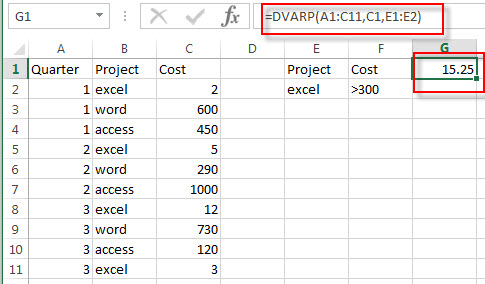### Related Functions

• Excel DVAR Function
The Excel DVAR Function will get the variance of a population based on a sample of numbers in a column in a list or database based on a given criteria.The syntax of the DVAR function is as below:= DVAR (database, field, criteria)…

## Excel DVAR Function

This post will guide you how to use Excel DVAR function with syntax and examples in Microsoft excel.

### Description

The Excel DVAR Function will get the variance of a population based on a sample of numbers in a column in a list or database based on a given criteria.

The DVAR function is a build-in function in Microsoft Excel and it is categorized as a Database Function.

The DVAR function is available in Excel 2016, Excel 2013, Excel 2010, Excel 2007, Excel 2003, Excel XP, Excel 2000, Excel 2011 for Mac.

### Syntax

The syntax of the DVAR function is as below:

`= DVAR (database, field, criteria)`

Where the DVAR function arguments are:

• Database -This is a required argument. The range of cells that containing the database.
• Field – This is a required argument. The column with database that you want the minimum of.
• Criteria – The range of cells that contains the conditions that you specify.

### Excel DVAR Function Examples

The below examples will show you how to use Excel DVAR Function to get the variance of a population based on a sample by using the numbers in a column in a list or database that match a given criteria.

#1 to get the sample variance of cost for the numbers in a range A1:C11,  using the following formula:

`=DVAR(A1:C11,C1,E1:E2)`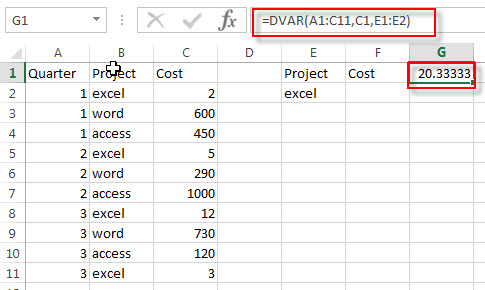### Related Functions

• Excel DVARP Function
The Excel DVARP Function will get the variance of a population based on the entire population of numbers in a column in a list or database based on a given criteria. The syntax of the DVARP function is as below:= DVARP(database, field, criteria)…

## Excel DSUM Function

This post will guide you how to use Excel DSUM function with syntax and examples in Microsoft excel.

### Description

The Excel DSUM Function will add the numbers in a column or database that meets a given criteria. And so you can use the DSUM function to add the numbers in a field of records in a list or database that match a given conditions that you specify in Excel.

The DSUM function is a build-in function in Microsoft Excel and it is categorized as a Database Function.

The DSUM function is available in Excel 2016, Excel 2013, Excel 2010, Excel 2007, Excel 2003, Excel XP, Excel 2000, Excel 2011 for Mac.

### Syntax

The syntax of the DSUM function is as below:

`= DSUM (database, field, criteria)`

Where the DSUM function arguments are:

• Database -This is a required argument. The range of cells that containing the database.
• Field – This is a required argument. The column with database that you want the minimum of.
• Criteria – The range of cells that contains the conditions that you specify.

### Excel DSUM Function Examples

The below examples will show you how to use Excel DSUM Function to get the sum of values from a set of records that match a given criteria.

#1 to get the total cost from excel project and its cost is greater than 200, using the following formula:

`=DSUM(A1:C10,C1,E1:F2)`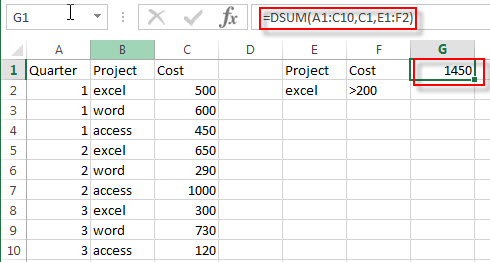## Excel DSTDEVP Function

This post will guide you how to use Excel DSTDEVP function with syntax and examples in Microsoft excel.

### Description

The Excel DSTDEVP returns the standard deviation of a population based on the entire population of numbers in a column or database that match the given criteria.

The DSTDEVP function is a build-in function in Microsoft Excel and it is categorized as a Database Function.

The DSTDEVP function is available in Excel 2016, Excel 2013, Excel 2010, Excel 2007, Excel 2003, Excel XP, Excel 2000, Excel 2011 for Mac.

### Syntax

The syntax of the DSTDEVP function is as below:

`=DSTDEVP(database, field, criteria)`

Where the DSTDEVP function arguments are:

• Database -This is a required argument. The range of cells that containing the database.
• Field – This is a required argument. The column with database that you want the minimum of.
• Criteria – The range of cells that contains the conditions that you specify.

### Excel DSTDEVP Function Examples

The below examples will show you how to use Excel DSTDEVP Function to calculate the standard deviation of population that matching the given criteria you specify.

#1 =DSTDEVP(A1:C10,C1,E1:F2)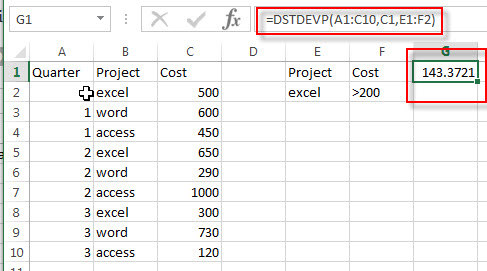### Related Functions

• Excel DSTDEV Function
The Excel DSTDEV returns the standard deviation of a population based on a sample of numbers in a column or database that match the given criteria.The syntax of the DSTDEV function is as below:= DSTDEV (database, field, criteria)…

## Excel DSTDEV Function

This post will guide you how to use Excel DSTDEV function with syntax and examples in Microsoft excel.

### Description

The Excel DSTDEV returns the standard deviation of a population based on a sample of numbers in a column or database that match the given criteria.

The DSTDEV function is a build-in function in Microsoft Excel and it is categorized as a Database Function.
The DSTDEV function is available in Excel 2016, Excel 2013, Excel 2010, Excel 2007, Excel 2003, Excel XP, Excel 2000, Excel 2011 for Mac.

### Syntax

The syntax of the DSTDEV function is as below:

`= DSTDEV (database, field, criteria)`

Where the DSTDEV function arguments are:

• Database -This is a required argument. The range of cells that containing the database.
• Field – This is a required argument. The column with database that you want the minimum of.
• Criteria – The range of cells that contains the conditions that you specify.

### Excel DSTDEV Function Examples

The below examples will show you how to use Excel DSTDEV Function to calculate the sample standard deviation of a column in a database or list that matching the given criteria you specify.

#1 =DSTDEV(A1:C10,C1,E1:F2)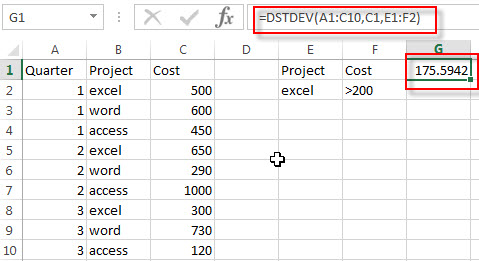### Related Functions

• Excel DSTDEVP Function
The Excel DSTDEVP returns the standard deviation of a population based on the entire population of numbers in a column or database that match the given criteria.The syntax of the DSTDEVP function is as below:=DSTDEVP(database, field, criteria)…

## Excel DMIN Function

This post will guide you how to use Excel DMIN function with syntax and examples in Microsoft excel.

### Description

The Excel DMIN returns the minimum value from a database or a column of a list that matches the specified conditions.

The DMIN function is a build-in function in Microsoft Excel and it is categorized as a Database Function.

The DMIN function is available in Excel 2016, Excel 2013, Excel 2010, Excel 2007, Excel 2003, Excel XP, Excel 2000, Excel 2011 for Mac.

### Syntax

The syntax of the DMIN function is as below:

`=DMIN(database, field, criteria)`

Where the DMIN function arguments are:

• Database -This is a required argument.  The range of cells that containing the database.
• Field – This is a required argument.  The column with database that you want the minimum of.
• Criteria – The range of cells that contains the conditions that you specify.

### Excel DMIN Function Examples

The below examples will show you how to use Excel DMIN Function to find the minimum value from a column of a list or database that matches a given criteria.

#1 = DMIN (A1:C10,C1,E1:F2)Note: The above excel formula will find excel project and its cost is greater than 200, then return the minimum one(300).

### Related Functions

• Excel Dmax Function
The Excel DMAX returns the maximum value from a database or a column of a list that matches the specified conditions.The syntax of the DMAX function is as below:= DMAX (database, field, criteria)…

## Excel DPRODUCT Function

This post will guide you how to use Excel DPRODUCT function with syntax and examples in Microsoft excel.

### Description

The Excel DPRODUCT returns the product of values from a set of records that match criteria that you specify.

The DPRODUCT function is a build-in function in Microsoft Excel and it is categorized as a Database Function.

The DPRODUCT function is available in Excel 2016, Excel 2013, Excel 2010, Excel 2007, Excel 2003, Excel XP, Excel 2000, Excel 2011 for Mac.

### Syntax

The syntax of the DPRODUCT function is as below:

`=DPRODUCT(database, field, criteria)`

Where the DPRODUCT function arguments are:

• Database -This is a required argument. The range of cells that containing the database.
• Field – This is a required argument. The column with database that you want the minimum of.
• Criteria – The range of cells that contains the conditions that you specify.

### Excel DPRODUCT Function Examples

The below examples will show you how to use Excel DPRODUCT Function to calculate the product of value from a column of a list or database that matches a given criteria.

#1 to get the product of the Cost from excel project with a cost is greater than 200, using the following formula:

`= DPRODUCT(A1:C10,C1,E1:F2)`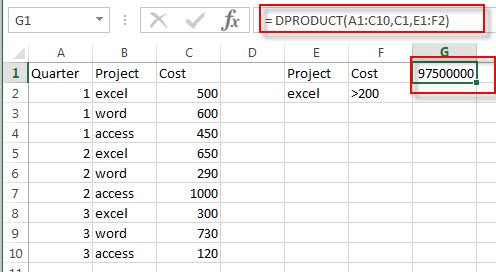## Excel Dmax Function

This post will guide you how to use Excel DMAX function with syntax and examples in Microsoft excel.

### Description

The Excel DMAX returns the maximum value from a database or a column of a list that matches the specified conditions.

The DMAX function is a build-in function in Microsoft Excel and it is categorized as a Database Function.

The DMAX function is available in Excel 2016, Excel 2013, Excel 2010, Excel 2007, Excel 2003, Excel XP, Excel 2000, Excel 2011 for Mac.

### Syntax

The syntax of the DMAX function is as below:

`= DMAX (database, field, criteria)`

Where the DMAX function arguments are:

• Database -This is a required argument.  The range of cells that containing the database.
• Field – This is a required argument.  The column with database that you want the maximum of.
• Criteria – The range of cells that contains the conditions that you specify.

### Excel DMAX Function Examples

The below examples will show you how to use Excel DMAX Function to find the maximum value from a column of a list or database that matches a given criteria.

#1 = DMAX (A1:C10,C1,E1:F2)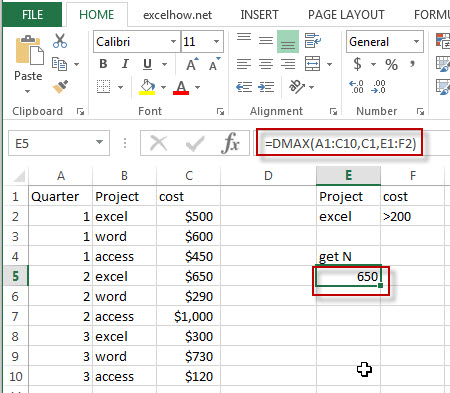Note: The above excel formula will find excel project and its cost is greater than 200, then return the maximum one(650).

Related Functions

• Excel DMIN Function
The Excel DMIN returns the minimum value from a database or a column of a list that matches the specified conditions.The syntax of the DMIN function is as below:=DMIN(database, field, criteria)…

## Excel Dget Function

This post will guide you how to use Excel DGET function with syntax and examples in Microsoft excel.

### Description

The Excel DGET returns a single value from a database that matches the specified conditions.

The DGET function is a build-in function in Microsoft Excel and it is categorized as a Database Function.

The DGET function is available in Excel 2016, Excel 2013, Excel 2010, Excel 2007, Excel 2003, Excel XP, Excel 2000, Excel 2011 for Mac.

### Syntax

The syntax of the DGET function is as below:

`= DGET(database, field, criteria)`

Where the DGET function arguments are:

• Database -This is a required argument.  The range of cells that containing the database.
• Field – This is a required argument.  The column to extract.
• Criteria – The range of cells that contains the conditions that you specify.

### Excel DGET Function Examples

The below examples will show you how to use Excel DGET Function to extract a single value from a column of a list or database that matches a given criteria.

#1 =DGET(A1:C10,C1,E1:F2)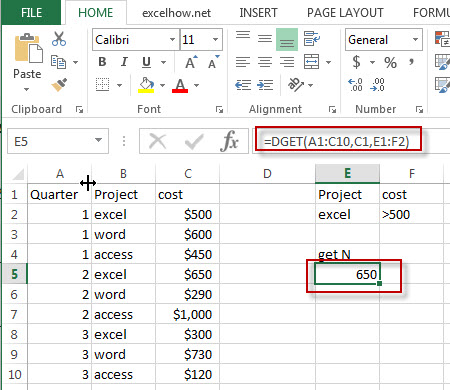Note: The above excel formula will find excel project and  its cost is greater than 500, then return this cost value.

## Excel DcountA Function

This post will guide you how to use Excel DCOUNTA function with syntax and examples in Microsoft excel.

### Description

The Excel DCOUNTA function returns the number of cells that containing nonblank values in a column of a list or a database that match the conditions that you specify.

The DCOUNTA function is a build-in function in Microsoft Excel and it is categorized as a Database Function.

The DCOUNTA function is available in Excel 2016, Excel 2013, Excel 2010, Excel 2007, Excel 2003, Excel XP, Excel 2000, Excel 2011 for Mac.

### Syntax

The syntax of the DCOUNTA function is as below:

`= DCOUNTA (database, field, criteria)`

Where the DCOUNTA function arguments are:

• Database -This is a required argument.  The range of cells that containing the database.
• Field – This is a required argument.  The column to count the values.
• Criteria – The range of cells that contains the conditions that you specify.

### Excel DCOUNTA Function Examples

The below examples will show you how to use Excel DCOUNTA Function to count the cells that contain nonblank values in a column of a list or database that match a given criteria.

#1 =DCOUNTA(A1:C10,C1,E1:F2)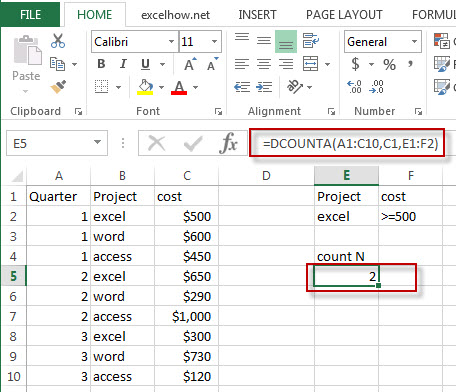Note: The above excel formula will find excel project and counts how many of the cost fields in those records contains non-blank values.

### Related Functions

• Excel Dcount Function
The Excel DCOUNT function returns the number of cells that containing numbers in a column of a list or a database that match the conditions that you specify.The syntax of the DCOUNT function is as below:= DCOUNT (database, field, criteria)…

## Excel Dcount Function

This post will guide you how to use Excel DCOUNT function with syntax and examples in Microsoft excel.

### Description

The Excel DCOUNT function returns the number of cells that containing numbers in a column of a list or a database that match the conditions that you specify.

The DCOUNT function is a build-in function in Microsoft Excel and it is categorized as a Database Function.

The DCOUNT function is available in Excel 2016, Excel 2013, Excel 2010, Excel 2007, Excel 2003, Excel XP, Excel 2000, Excel 2011 for Mac.

### Syntax

The syntax of the DCOUNT function is as below:

`= DCOUNT (database, field, criteria)`

Where the DCOUNT function arguments are:

• Database -This is a required argument.  The range of cells that containing the database.
• Field – This is a required argument.  The column to count the values.
• Criteria – The range of cells that contains the conditions that you specify.

### Excel DCOUNT Function Examples

The below examples will show you how to use Excel DCOUNT Function to count the cells that contain numbers in a column of a list or database that match a given criteria.

#1 =DCOUNT(A1:C10,C1,E1:F2)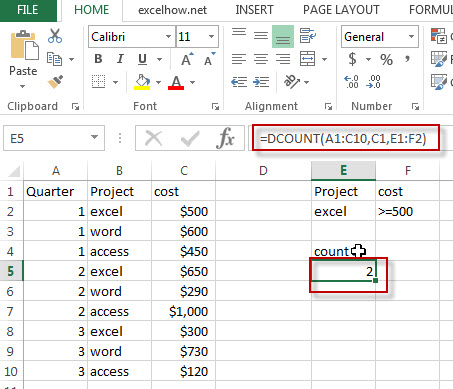Note: The above excel formula will find excel project and counts how many of the cost fields in those records contains numbers.

### Related Functions

• Excel DcountA Function
The Excel DCOUNTA function returns the number of cells that containing nonblank values in a column of a list or a database that match the conditions that you specify.The syntax of the DCOUNTA function is as below:= DCOUNTA (database, field, criteria)…

## Excel Daverage Function

This post will guide you how to use Excel DAVERAGE function with syntax and examples in Microsoft excel.

### Description

The Excel DAVERAGE function calculates the average of values in a column of a list or database that match conditions you specify.

The DAVERAGE function is a build-in function in Microsoft Excel and it is categorized as a Database Function.

The DAVERAGE function is available in Excel 2016, Excel 2013, Excel 2010, Excel 2007, Excel 2003, Excel XP, Excel 2000, Excel 2011 for Mac.

### Syntax

The syntax of the DAVERAGE function is as below:

`= DAVERAGE(database, field, criteria)`

Where the DAVERAGE function arguments are:

• Database -This is a required argument.  The range of cells that containing the database.
• Field – This is a required argument.  The column to average the values.
• Criteria – The range of cells that contains the conditions that you specify.

### Excel DAVERAGE Function Examples

The below examples will show you how to use Excel DAVERAGE Function to average all numbers in a column of a list or database based on a given criteria.

#1 =DAVERAGE(A1:C10,C1,E1:E2)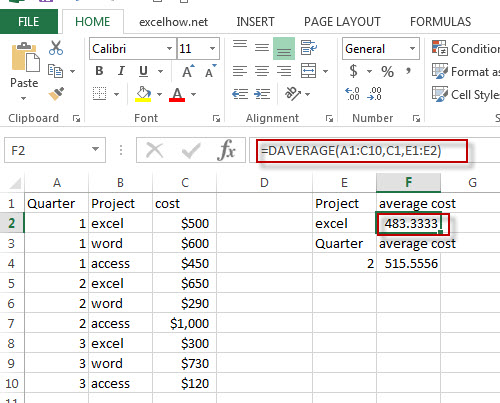Note: The above formula will return the average cost of project excel in the three quarter.